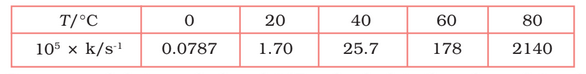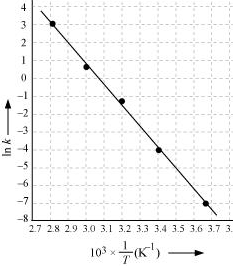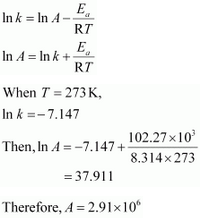# 4.22   The rate constant for the decomposition of N2O5 at various temperatures              is given below:Draw a graph between ln k and 1/T and calculate the values of A and             . Predict the rate constant at 30° and 50°C.

M manish

From the above data,

 T/ 0 20 40 60 80 T/K 273 293 313 333 353 () 3.66 3.41 3.19 3 2.83 0.0787 1.7 25.7 178 2140 -7.147 -4.075 -1.359 -0.577 3.063Slope of line  =

According to Arrhenius equations,

Slope =
12.30 8.314
= 102.27

Again,When T = 30 +273 = 303 K and 1/T =0.0033K

k =

When T = 50  + 273 = 323 K  and 1/T = 3.1  K

k = 0.607 per sec

Exams
Articles
Questions